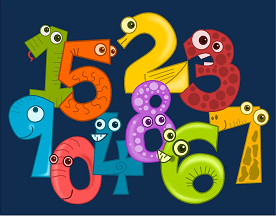Fear of working with numbers is a major problem of a large number of students considered to be suffering from Numerophobia or Arithmophobia . On checking Accountancy examination papers, I observe so called silly mistakes students commit . Many students end up with far less marks than their expectation simply  because of incorrect calculations.

Commerce students must understand that one calculation mistake leads to another in another account thus mismatch of Balance Sheet, the ultimate target to be achieved by them in most of the questions. I tried to find out ,why students do so .

Then I thought of writing something to make them interested in dealing with mathematical figures . When, I just started doing it on my own cell phone calculator, I was excited with the beauty of the numbers. I just spent some time, you can try more………

## Experiment 1

1=1

1+11=12

1+11+111=123

1+11+111+1111=1234

1+11+111+1111+11111=12345

Try it further……….

## Experiment 2

1×1=1

11×11=121

111×111=12321

1111×1111=1234321

11111×11111=123454321

Try it further…………

## Experiment 3

1+9=10(Total of digits=1)

11+90=101(Total of digits=2)

111+900=1011(Total of digits=3)

1111+9000=10111(Total of digits=4)

11111+90000=101111(Total of digits=5)

Try it further………..

## Experiment  4

1×1=1(1)

2×2=4(1+2+1)

3×3=9(1+2+3+2+1)

4×4=16(1+2+3+4+3+2+1)

5×5=25(1+2+3+4+5+4+3+2+1)

Try it further………….

## Experiment  5

1×1=1

11×11=121

111×111=12321

1111×1111=1234321

…………………………….

111111111×111111111=12345678987654321

You may try further……..

Now, I understand  how  the following  relations were found by some people out there.

### Expressing the total of 100 using digits 1 to 9

123 – 45 – 67 + 89 = 100.

123 + 4 – 5 + 67 – 89 = 100.

123 – 4 – 5 – 6 – 7 + 8 – 9 = 100.

1 + 23 – 4 + 5 + 6 + 78 – 9 = 100.

You may try further…………..

Similarly, starting from digit 9……..

98 + 7 – 6 + 5 – 4 + 3 – 2 – 1 = 100
98 + 7 – 6 + 5 – 4 – 3 + 2 + 1 = 100
98 – 7 + 6 + 5 – 4 + 3 – 2 + 1 = 100
98 – 7 + 6 – 5 + 4 + 3 + 2 – 1 = 100

You may try further…………..

Just because they were attracted towards the Beauty of Numbers.

I also understand the reality of ‘The Human Computer’  the famous Indian Shakuntala Devi who had defeated a computer in U.S.  for calculating the cube root of 18,81,38,517, in 1971. She calculated it faster than it could be done on computer.

Quick Tips for Calculations